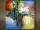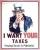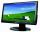Three workers

Three workers got paid for work. First earn € 50, the second € 100 and third € 60. For further work should receive together € 735. How do you divide that amount in order to be distributed in the same proportion as the first job?

Result

a =  175 Eur
b =  350 Eur
c =  210 Eur

Solution:Leave us a comment of this math problem and its solution (i.e. if it is still somewhat unclear...):Be the first to comment!Next similar math problems:

1. RunnersFor three runners (on the first to third place) is prepared 30 chocolate that they be distributed in the ratio of 3 : 2 : 1 How much chocolate will get everyone?
2. Three 43Three brothers inherited a cash amount of 62,000 and they divided it among themselves in the ratio of 5:4:1. How much more is the largest share than the smallest share?
3. Hour salaryYou work for 4 hours on a Saturday and 8 hours on Sunday. You also receive a \$50 bonus. You earn \$164. How much did you earn per hour?
4. BicycleThe bicycle pedal gear has 36 teeth, the rear gear wheel has 10 teeth. How many times turns rear wheel, when pedal wheel turns 120x?
5. DonutsFind how many donuts each student will receive if you share 126 donuts in a ratio of 1:5:8
6. Image scaleThe actual image dimensions are 60 cm x 80cm and has a reduced size 3 cm x 4 cm. At what scale the image was reduced?
7. Line segmentThe 4 cm long line segment is enlarged in the ratio of 5/2. How many centimeters will measure the new line segment?
8. PeppersIn the box are yellow (a), green (b) and red (c) peppers. Their amount is in a ratio 2:4:1 . Most are yellow peppers and green the least. Calculate the number of peppers each type if the total number of peppers is 70.
9. AgesJohn, Teresa, Daniel and Paul have summary 56 years. Their ages are in a ratio of 1:2:5:6. Determine how many years have each of them.
10. Land areaA land area of Asia and Africa are in a 3: 2 ratio, the European and African are is 1:3. What are the proportions of Asia, Africa, and Europe?
11. Moneys in triple ratioMilan, John and Lili have a total 344 euros. Their amounts are in the ratio 1:2:5. Determine how much each of them has?
12. VATVAT is a tax which the state artificially betrays goods and services for final consumption. VAT in Slovakia is 20%. Calculate how much percent pay less tax residents of Liechtenstein, when VAT is only 8%.
13. Arble bagA marble bag sold by Rachel's Marble Company contains 5 orange marbles for every 6 green marbles. If a bag has 35 orange marbles, how many green marbles does it contain?
14. Washing machineThe price of washing machine was decreased by 21% and then by 55 € due small sales. After two price decreases cost € 343. How much was originally cost?
15. 1.5 divided1.5 divided by 1 = w divided by 4
16. Masons1 mason casts 30.8 meters square in 8 hours. How long casts 4 masons 178 meters square?
17. Sales off 2Computer monitor sold for 8400 CZK in March. In April, dealer price decreased by 798 CZK. Calculate the percentage by which the price was reduced.